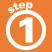Home    |    Teacher    |    Parents    |    Glossary    |    About UsTo multiply fractions:

1. Simplify the fractions if not in lowest terms.
2. Multiply the numerators of the fractions to get the new numerator.
3. Multiply the denominators of the fractions to get the new denominator.

Simplify the resulting fraction if possible.

Click on the example.

 Homework Help | Pre-Algebra | FractionsEmail this page to a friendSearch·  Definitions·  Reducing fractions·  Adding and subtract-     ing fractions·  Multiplying fractions·  Dividing fractions·  Adding and subtract-     ing mixed numbers·  Multiplying     mixed numbers·  Dividing     mixed numbersFirst Glance In Depth Examples WorkoutMultiplying fractions